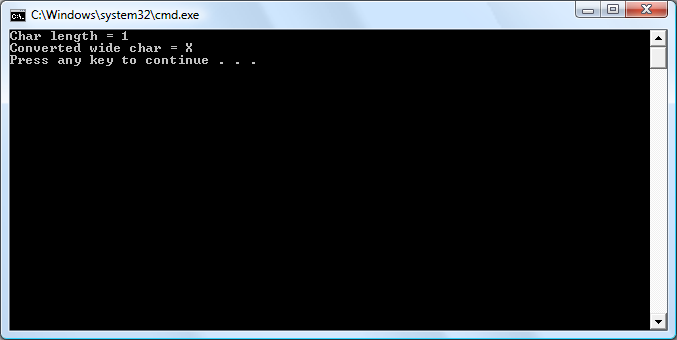• Programming
• Internet

# mbrtowc()

### Declaration

```size_t mbrtowc(wchar_t* wpConverted,
const char* kcpMChar,
size_t qMaxSize,
mbstate_t qMBState);```

### Description

This function converts the multibyte character that "kcpMChar" points into a wide char and puts it into the wchar_t pointed to by "wpConverted." The function checks "qMaxSize" bytes after "kcpMChar" to find the end of the entire multibyte character an returns the number of bytes remaining in the multibyte character, using "qMBState" as the multibyte shift state. If "qMBState" is NULL, then the internal shift state is used.

The call can fail for several reasons and will return the following:

0     if "kcpMChar" points to the NULL wide character
-1     if "kcpMChar" does not point to a valid multibyte character
-2     if "qMaxSize" is not large enough to contain the character

### Example

```#include <iostream>
#include <cwchar>

int main()
{
using namespace std;
char* cpName = "XoaX.txt";
wchar_t wWideChar;

// Convert the first character of cpName
size_t qReturn = mbrtowc(&wWideChar, cpName, MB_CUR_MAX, NULL);
// Check whether the call was successful.
if (qReturn < 0) {
cout << "Error!" << endl;
} else {
cout << "Char length = " << qReturn << endl;
wcout << "Converted wide char = " << wWideChar << endl;
}
return 0;
}```

### Output